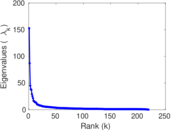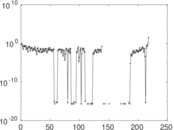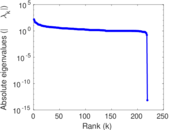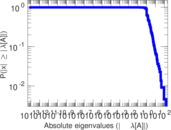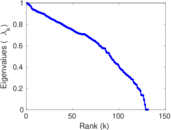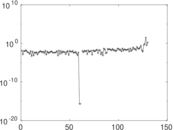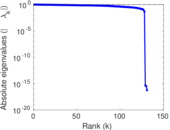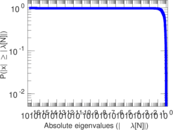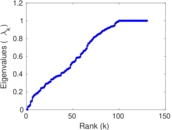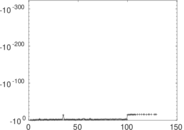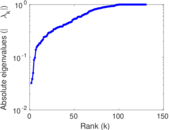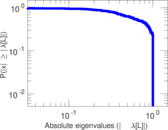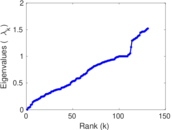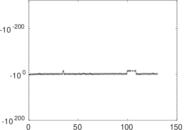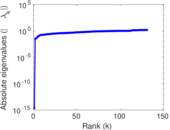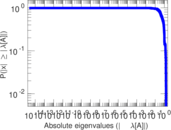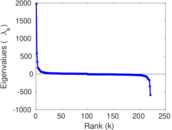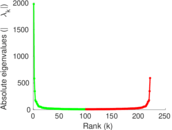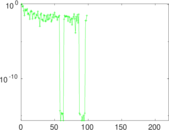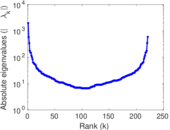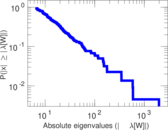# Wikiquote edits (lt)

This is the bipartite edit network of the Lithuanian Wikisource. It contains users and pages from the Lithuanian Wikisource, connected by edit events. Each edge represents an edit. The dataset includes the timestamp of each edit.

 Code `qlt` Internal name `edit-ltwikisource` Name Wikiquote edits (lt) Data source http://dumps.wikimedia.org/ AvailabilityDataset is available for download Consistency checkDataset passed all tests Category Authorship network Dataset timestamp 2017-10-20 Node meaning User, article Edge meaning Edit Network formatBipartite, undirected Edge typeUnweighted, multiple edges Temporal dataEdges are annotated with timestamps

## Statistics

 Size n = 1,983 Left size n1 = 221 Right size n2 = 1,762 Volume m = 4,944 Unique edge count m̿ = 2,672 Wedge count s = 332,432 Claw count z = 62,710,067 Cross count x = 10,502,052,539 Square count q = 13,361 4-Tour count T4 = 1,442,740 Maximum degree dmax = 1,778 Maximum left degree d1max = 1,778 Maximum right degree d2max = 95 Average degree d = 4.986 38 Average left degree d1 = 22.371 0 Average right degree d2 = 2.805 90 Fill p = 0.006 861 80 Average edge multiplicity m̃ = 1.850 30 Size of LCC N = 1,741 Diameter δ = 14 50-Percentile effective diameter δ0.5 = 3.633 01 90-Percentile effective diameter δ0.9 = 6.690 36 Median distance δM = 4 Mean distance δm = 4.410 91 Gini coefficient G = 0.725 704 Balanced inequality ratio P = 0.214 300 Left balanced inequality ratio P1 = 0.123 989 Right balanced inequality ratio P2 = 0.307 443 Relative edge distribution entropy Her = 0.799 981 Power law exponent γ = 3.822 15 Tail power law exponent γt = 2.341 00 Tail power law exponent with p γ3 = 2.341 00 p-value p = 0.000 00 Left tail power law exponent with p γ3,1 = 1.711 00 Left p-value p1 = 0.427 000 Right tail power law exponent with p γ3,2 = 3.661 00 Right p-value p2 = 0.173 000 Degree assortativity ρ = −0.249 760 Degree assortativity p-value pρ = 2.782 40 × 10−39 Spectral norm α = 152.636 Algebraic connectivity a = 0.032 350 4 Spectral separation |λ1[A] / λ2[A]| = 1.754 95 Controllability C = 1,540 Relative controllability Cr = 0.779 352

## Plots

### Fruchterman–Reingold graph drawing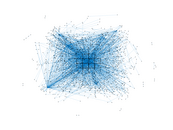### Degree distribution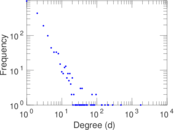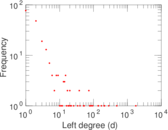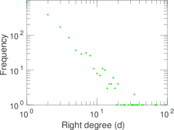### Cumulative degree distribution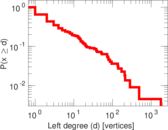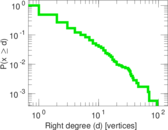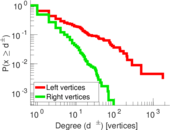### Lorenz curve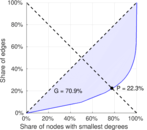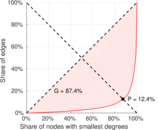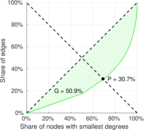### Spectral distribution of the adjacency matrix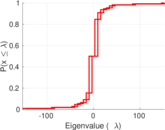### Spectral distribution of the normalized adjacency matrix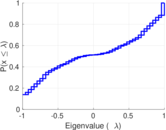### Spectral distribution of the Laplacian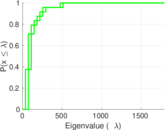### Spectral graph drawing based on the adjacency matrix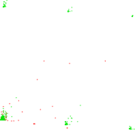### Spectral graph drawing based on the Laplacian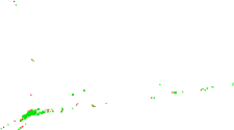### Spectral graph drawing based on the normalized adjacency matrix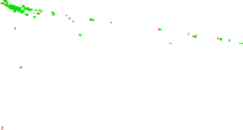### Degree assortativity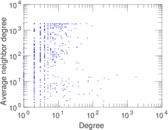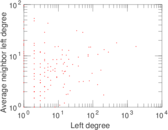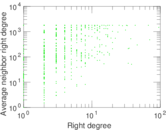### Zipf plot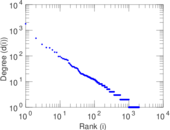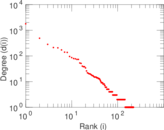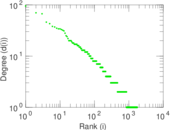### Hop distribution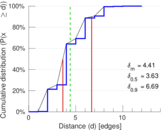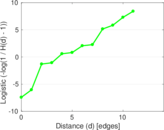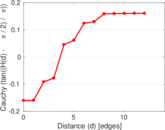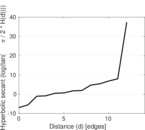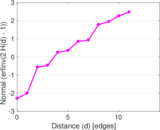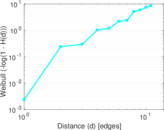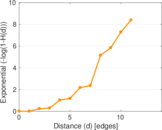### Double Laplacian graph drawing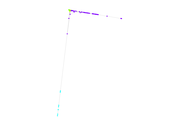### Delaunay graph drawing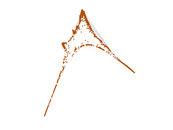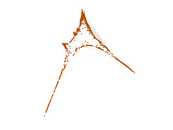### Edge weight/multiplicity distribution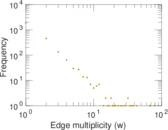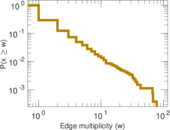### Temporal distribution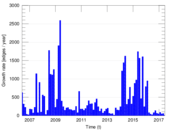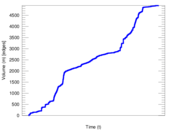### Temporal hop distribution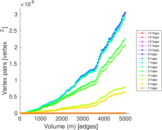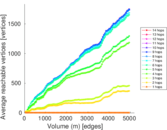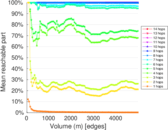### Diameter/density evolution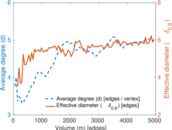### Matrix decompositions plots